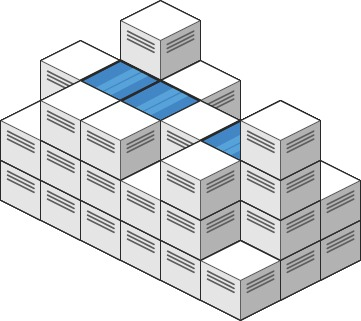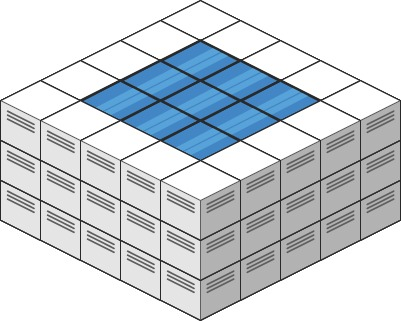### LeetCode• ㊗️
• 大家
• offer
• 多多！

## Problem

Given an m x n integer matrix heightMap representing the height of each unit cell in a 2D elevation map, return the volume of water it can trap after raining.

Example 1:Input: heightMap = [[1,4,3,1,3,2],[3,2,1,3,2,4],[2,3,3,2,3,1]]
Output: 4
Explanation: After the rain, water is trapped between the blocks.
We have two small ponds 1 and 3 units trapped.
The total volume of water trapped is 4.


Example 2:Input: heightMap = [[3,3,3,3,3],[3,2,2,2,3],[3,2,1,2,3],[3,2,2,2,3],[3,3,3,3,3]]
Output: 10


## Code

class Solution {

public class Cell {
int row;
int col;
int height;
public Cell(int row, int col, int height) {
this.row = row;
this.col = col;
this.height = height;
}
}

public int trapRainWater(int[][] heights) {
if (heights == null || heights.length == 0 || heights.length == 0)
return 0;

PriorityQueue<Cell> queue = new PriorityQueue<>(1, new Comparator<Cell>(){
public int compare(Cell a, Cell b) {
return a.height - b.height;
}
});

int m = heights.length;
int n = heights.length;
boolean[][] visited = new boolean[m][n];

// Initially, add all the Cells which are on borders to the queue.
for (int i = 0; i < m; i++) {
visited[i] = true;
visited[i][n - 1] = true;
queue.offer(new Cell(i, 0, heights[i]));
queue.offer(new Cell(i, n - 1, heights[i][n - 1]));
}

for (int i = 0; i < n; i++) {
visited[i] = true;
visited[m - 1][i] = true;
queue.offer(new Cell(0, i, heights[i]));
queue.offer(new Cell(m - 1, i, heights[m - 1][i]));
}

// from the borders, pick the shortest cell visited and check its neighbors:
// if the neighbor is shorter, collect the water it can trap and update its height as its height plus the water trapped
// add all its neighbors to the queue.
int[][] dirs = new int[][]{{-1, 0}, {1, 0}, {0, -1}, {0, 1}};
int res = 0;
while (!queue.isEmpty()) {
Cell cell = queue.poll();
for (int[] dir : dirs) {
int row = cell.row + dir;
int col = cell.col + dir;
if (row >= 0 && row < m && col >= 0 && col < n && !visited[row][col]) {
visited[row][col] = true;
res += Math.max(0, cell.height - heights[row][col]);
queue.offer(new Cell(row, col, Math.max(heights[row][col], cell.height)));
}
}
}

return res;
}
}